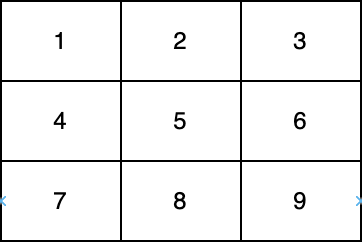2614. Prime In Diagonal
Easy
220
24

You are given a 0-indexed two-dimensional integer array `nums`.

Return the largest prime number that lies on at least one of the diagonals of `nums`. In case, no prime is present on any of the diagonals, return 0.

Note that:

• An integer is prime if it is greater than `1` and has no positive integer divisors other than `1` and itself.
• An integer `val` is on one of the diagonals of `nums` if there exists an integer `i` for which `nums[i][i] = val` or an `i` for which `nums[i][nums.length - i - 1] = val`.In the above diagram, one diagonal is [1,5,9] and another diagonal is [3,5,7].

Example 1:

```Input: nums = [[1,2,3],[5,6,7],[9,10,11]]
Output: 11
Explanation: The numbers 1, 3, 6, 9, and 11 are the only numbers present on at least one of the diagonals. Since 11 is the largest prime, we return 11.
```

Example 2:

```Input: nums = [[1,2,3],[5,17,7],[9,11,10]]
Output: 17
Explanation: The numbers 1, 3, 9, 10, and 17 are all present on at least one of the diagonals. 17 is the largest prime, so we return 17.
```

Constraints:

• `1 <= nums.length <= 300`
• `nums.length == numsi.length`
• `1 <= nums[i][j] <= 4*106`
Accepted
25.7K
Submissions
79.3K
Acceptance Rate
32.5%

Seen this question in a real interview before?
1/4
Yes
No

Discussion (0)

Related Topics